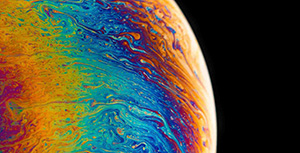• 累计撰写 247 篇文章
• 累计创建 16 个标签
• 累计收到 0 条评论

### 目 录CONTENT# TensorFlow 2 深度神经网络2021-09-23 / 0 评论 / 0 点赞 / 117 阅读 / 8,044 字

### VGG 网络结构

VGG 是 Visual Geometry Group 的简称，前者代表牛津大学工程科学系的视觉几何课题组。2014 年，VGG 在 ImageNet ILSVRC-2014 分类任务中拿下了第二名的成绩。VGG 的特点在于卷积层和池化层均采用相同的卷积核（3 \times 33×3）和池化核参数（2 \times 22×2）。同时，网络由多个卷积池化单元块组成，具体来说就是若干个卷积层加上一个池化层组合。

### VGG 模型构建

import tensorflow as tf

model_vgg = tf.keras.Sequential([
tf.keras.layers.Conv2D(64, (3, 3), input_shape=(224, 224, 3), padding='same', activation='relu'),
tf.keras.layers.Conv2D(64, (3, 3), activation='relu', padding='same'),
tf.keras.layers.MaxPooling2D(pool_size=(2, 2), strides=(2, 2)),
tf.keras.layers.Conv2D(128, (3, 3), activation='relu', padding='same'),
tf.keras.layers.Conv2D(128, (3, 3), activation='relu', padding='same',),
tf.keras.layers.MaxPooling2D(pool_size=(2, 2), strides=(2, 2)),
tf.keras.layers.Conv2D(256, (3, 3), activation='relu', padding='same',),
tf.keras.layers.Conv2D(256, (3, 3), activation='relu', padding='same',),
tf.keras.layers.Conv2D(256, (3, 3), activation='relu', padding='same',),
tf.keras.layers.MaxPooling2D(pool_size=(2, 2), strides=(2, 2)),
tf.keras.layers.Conv2D(512, (3, 3), activation='relu', padding='same',),
tf.keras.layers.Conv2D(512, (3, 3), activation='relu', padding='same',),
tf.keras.layers.Conv2D(512, (3, 3), activation='relu', padding='same',),
tf.keras.layers.MaxPooling2D(pool_size=(2, 2), strides=(2, 2)),
tf.keras.layers.Conv2D(512, (3, 3), activation='relu', padding='same',),
tf.keras.layers.Conv2D(512, (3, 3), activation='relu', padding='same',),
tf.keras.layers.Conv2D(512, (3, 3), activation='relu', padding='same',),
tf.keras.layers.MaxPooling2D(pool_size=(2, 2), strides=(2, 2)),
tf.keras.layers.Flatten(),
tf.keras.layers.Dense(4096, activation='relu'),
tf.keras.layers.Dense(4096, activation='relu'),
tf.keras.layers.Dense(1000, activation='softmax')
])

model_vgg.summary()### Fashion-MNIST 分类

VGG 本身是为 ImageNet 分类任务设计，ImageNet 是一个包含数万张图片的大型基准数据集，总共有 1000 个目标输出。所以，你也可以看出 VGG16 最终输出层为 1000。不过，由于 ImageNet 数据集体积太大，本次实验我们选择一个较小的 Fashion-MNIST 时尚物品分类数据集。

Fashion-MNIST 时尚物品数据集包含 70,000 张图片，其中训练集为 60,000 张 28x28 像素灰度图像，测试集为 10,000 同规格图像，总共 10 类时尚物品标签。wget -nc "http://labfile.oss.aliyuncs.com/courses/1211/fashion-mnist.zip"
mkdir -p "/root/.keras/datasets/fashion-mnist/"
unzip -o "fashion-mnist.zip" -d "/root/.keras/datasets/fashion-mnist"


import tensorflow as tf
import numpy as np

(X_train, y_train), (X_test, y_test) = tf.keras.datasets.fashion_mnist.load_data()

# 对特征进行归一化处理
X_train = X_train / 255
X_test = X_test / 255
# 对标签进行独热编码
y_train = np.eye(10)[y_train]
y_test = np.eye(10)[y_test]

X_train.shape, X_test.shape, y_train.shape, y_test.shape


from matplotlib import pyplot as plt
%matplotlib inline

plt.imshow(X_train, cmap=plt.cm.gray)


#### 数据不变，修改网络

model_mnist = tf.keras.Sequential([
tf.keras.layers.Conv2D(64, (3, 3), input_shape=(28, 28, 1), padding='same', activation='relu'),
tf.keras.layers.Conv2D(64, (3, 3), activation='relu', padding='same'),
tf.keras.layers.MaxPooling2D(pool_size=(2, 2), strides=(2, 2)),
tf.keras.layers.Conv2D(128, (3, 3), activation='relu', padding='same'),
tf.keras.layers.Conv2D(128, (3, 3), activation='relu', padding='same',),
tf.keras.layers.MaxPooling2D(pool_size=(2, 2), strides=(2, 2)),
tf.keras.layers.Conv2D(256, (3, 3), activation='relu', padding='same',),
tf.keras.layers.Conv2D(256, (3, 3), activation='relu', padding='same',),
tf.keras.layers.Conv2D(256, (3, 3), activation='relu', padding='same',),
tf.keras.layers.MaxPooling2D(pool_size=(2, 2), strides=(2, 2)),
tf.keras.layers.Flatten(),
tf.keras.layers.Dense(4096, activation='relu'),
tf.keras.layers.Dense(4096, activation='relu'),
tf.keras.layers.Dense(10, activation='softmax')
])

model_mnist.summary()


# 对图像形状进行处理，增加一个通道维度
X_train = tf.reshape(X_train, [-1, 28, 28, 1])
X_test = tf.reshape(X_test, [-1, 28, 28, 1])

X_train.shape, X_test.shape, y_train.shape, y_test.shape

# 编译模型
loss='categorical_crossentropy', metrics=['accuracy'])
# 训练模型
history = model_mnist.fit(X_train, y_train, epochs=3, batch_size=32,
validation_data=(X_test, y_test))


#### 网络不变，修改数据

# 对图像进行 Resize 操作，变为 224 尺寸
X_train_demo = tf.image.resize(X_train[:100], (224, 224))
X_test_demo = tf.image.resize(X_test[:100], (224, 224))
# 将单通道灰度图像转换为 3 通道 RGB 图像
X_train_demo = tf.image.grayscale_to_rgb(X_train_demo)
X_test_demo = tf.image.grayscale_to_rgb(X_test_demo)

X_train_demo.shape, X_test_demo.shape


mnist_out = tf.keras.layers.Dense(10, activation='softmax')(model_vgg.layers[-2].output)
mnist_model_vgg = tf.keras.Model(model_vgg.input, mnist_out)
mnist_model_vgg.summary()


### VGG 迁移学习

TensorFlow 提供了多种卷积神经网络在大规模数据上训练好的模型，你可以很轻松找到 VGG16 在 ImageNet 上的预训练模型。接下来，我们尝试加载此模型：

wget -nc "http://labfile.oss.aliyuncs.com/courses/1282/vgg16_weights.zip"
unzip -o "vgg16_weights.zip" -d "/root/.keras/models"

vgg16_notop = tf.keras.applications.VGG16(include_top=False, input_shape=(32, 32, 3))
vgg16_notop.summary()


flatten = tf.keras.layers.Flatten()(vgg16_notop.output) # 将模型 Flatten 展平
outs = tf.keras.layers.Dense(10, activation='softmax')(flatten) # 连接到 10 输出
model_pretrained = tf.keras.models.Model(inputs=vgg16_notop.input, outputs=outs) # 组合为新模型
model_pretrained.summary()


for layer in model_pretrained.layers[:-2]:
layer.trainable = False  # 固定前面层参数
for layer in model_pretrained.layers[-2:]:
layer.trainable = True  # 后两层可训练
# 查看修改后每层可训练状态
for layer in model_pretrained.layers:
print(layer.name, layer.trainable)


# 对图像进行 Resize 操作，变为 224 尺寸
X_train_lite = tf.image.resize(X_train[:10000], (32, 32))
X_test_lite = tf.image.resize(X_test, (32, 32))
# 将单通道灰度图像转换为 3 通道 RGB 图像
X_train_lite = tf.image.grayscale_to_rgb(X_train_lite)
X_test_lite = tf.image.grayscale_to_rgb(X_test_lite)

y_train_lite = y_train[:10000]
y_test_lite = y_test

X_train_lite.shape, X_test_lite.shape, y_train_lite.shape, y_test_lite.shape


# 编译模型
loss='categorical_crossentropy', metrics=['accuracy'])
# 训练模型
history = model_pretrained.fit(X_train_lite, y_train_lite, epochs=10, batch_size=32,
validation_data=(X_test_lite, y_test_lite))


0
1.2.• 0
1.2.